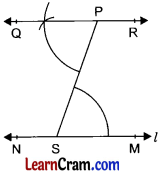# DAV Class 6 Maths Chapter 14 Brain Teasers Solutions

The DAV Class 6 Maths Book Solutions and DAV Class 6 Maths Chapter 14 Brain Teasers Solutions of Constructions offer comprehensive answers to textbook questions.

## DAV Class 6 Maths Ch 14 Brain Teasers Solutions

Question 1.
A. Tick (✓) the correct answer.
(a) Which of the following divides a line segment into two equal parts?
(i) perpendicular at any point.
(ii) perpendicular bisector of the segment
(iii) angle bisector
(iv) both (ii) and (iii)
(ii) perpendicular bisector of the segment.
Perpendicular bisector of a line segment divides the line into two equal parts.

(b) Two lines perpendicular to the same line will always
(i) intersecting each other
(ii) be concurrent lines
(iii) be parallel to each other
(iv) overlap each other
(iii) be parallel to each other
Two lines perpendicular on the same line are parallel to each other.

(c) The angle that cannot be constructed using ruler and compasses, is:
(i) 30°
(ii) 45°
(iii) 75°
(iv) 70°
(iv) 70°
All the angles like 30°, 45° and 75° can be constructed by using ruler and compass but 70° cannot be formed with this process.

(d) In the given figure, bisector CF of ∠DCE will make ∠BCF equal to: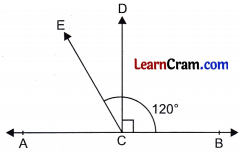(i) 100°
(ii) 105°
(iii) 110°
(iv) 100.5°
(ii) 105°
∠BCE = 120°
⇒ ∠BCD + ∠DCE = 120°
⇒ 90° + ∠DCE = 120°
Now, ∠DCF = $$\frac{1}{2}$$ × ∠DCE
= $$\frac{1}{2}$$ × 30° = 15°
∠BCF = ∠BCD + ∠DCF
= 90° + 15°
= 105°

(e) We get the angle of measure 11.25° by bisecting the angle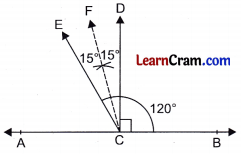(i) 45°
(ii) 22°
(iii) 40°
(iv) 22.5°
(iv) 22.5°
$$\frac{1}{2}$$ × 22.5°
= 11.25°Question 2.
Draw a line m. From a point X outside the line, construct a perpendicular to line m using compasses and ruler.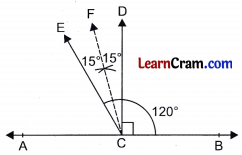Step 1 : Draw a line rn and any point X outside the line rn.
Step 2: Taking X as centre and suitable radius draw an arc to intersect the line m at A and B.
Step 3: Taking A and B as centre and suitable radius, draw two arcs to intersect each other at C.
Step 4: Join XC and produce to D which intersects line m at Y. Then XY is the required perpendicular from X to line m.

Question 3.
Construct the following angles using compasses and ruler.
(a) 120°Step 1: Draw a ray AB.
Step 2: Take A as centre and suitable radius, draw an arc intersecting AB at D.
Step 3: Taking D as centre and same radius taken in step 2, draw two continuous arcs intersecting the first arc at E and F.
Step 4: Join A and F and produce to C.
Then ∠CAB = 120°.

(b) 90°Draw a ray AB.
Take A as centre and suitable radius, draw an arc intersecting AB at D.
Taking D as centre and same radius, draw two continuous arcs at E and F.
Taking E and F as centres and suitable radius, draw two arcs which intersect each other at G.
Join AG and produce to C.
Then ∠CAB = 90°.

(c) 30°Draw a ray AB.
Take A as centre and suitable radius, draw an arc intersecting AB at D.
Take D as centre and with same radius, draw an arc intersecting the first arc at E.
Take D and E as the centre and with same radius, draw two arcs intersecting each other at F.
Join AF and produce to C.
Then ∠CAB = 30°

(d) 15°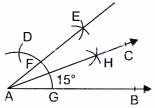Draw a ray AB.
Take A as centre and suitable radius, draw an arc intersecting AB at G.
Take G as centre and with the same radius, draw an arc which intersects the first arc at D. A G B
Draw two arcs taking G and D as centres intersecting each other at E. Join AE which intersects the first arc at F.
Draw two arcs taking G and F as centre with suitable radius which intersects each other at H.
Join AH and produce to C.
Then ∠CAB = 15°.

(e) 180°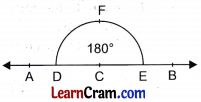Step 1 : Draw a line AB and take any point C on it.
Step 2: Take C as centre and with suitable radius, draw a semi-circle which meets the line AB at D and E.
Then ∠DFE = 180°Question 4.
Draw a line AB equal to 10 cm. Mark a point X such that AX is equal to 6 cm. Draw line through X perpendicular to AB using compass and ruler.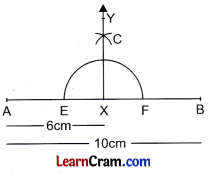Step 1: Draw AB = 10 cm.
Step 2: Make AX = 6 cm with the help of compass.
Step 3: Take X as centre and with suitable radius, draw an arc which meets the line at E and F.
Step 4: Draw two arcs with centres E and F, these two arcs intersecting each other at C.
Step 5: Join XC and produce to Y.
Then XY is the required perpendicular to AB.

Question 5.
Draw an angle PQR of any measure. Now using compass, draw another ∠XYZ equal to ∠PQR.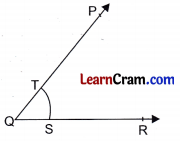Step 1: Draw ∠PQR of any measure.
Step 2: Take Q as centre and draw an arc ST.
Step 3: Draw a ray YZ.
Step 4: Take Y as centre and with suitable radius, draw an arc which meets YZ at W.
Step 5: Draw an arc with centre W and radius ST which intersects the first arc at T.
Step 6: Join YT and produce to X.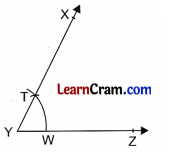Then ∠XYZ = ∠PQR.

Question 6.
Draw a circle of radius 4 cm and centre O. Draw any chord and name it AB. Using compasses, draw the perpendicular from the centre O to the chord AB.Step 1: Draw a circle with radius 4 cm.
Step 2: Draw a chord AB.
Step 3: Take O as centre and draw an arc with suitable radius intersecting the chord AB at E and F.
Step 4: Taking E and F centres and with suitable radius, draw two arcs which intersect each other at D.
Step 5: Join OD to meet AB at C. Then OC is perpendicular to chord AB.

### DAV Class 6 Maths Chapter 14 HOTS

Question 1.
Construct an angle ∠ABC of 150° using ruler and compasses and draw the angle bisector BD of ∠ABC. Mark points P, Q, R in the angular region of the bisector of angle 150°, i.e., ∠ABD. Also mark points S, T, U in its exterior.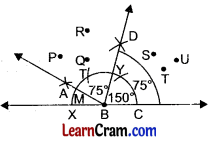Steps of Construction
(i) Draw a line segment XC.
(ii) With B as centre and with any radius, draw a semicircle on upper side of the line segment,
(iii) With C as centre and with the same radius, draw arc at Y.
(iv) With Y as centre and with the same radius, draw arc at T.
(v) Now with X and T as centres and radius equal to more than $$\frac{1}{2}$$XT, draw arcs which cut each other at A.
(vi) Join A to B
Thus, ABC = ∠150°
(vii) Now with C and M as centres and radius equal to more than $$\frac{1}{2}$$MC, draw arcs which cut each other at D.
Now, ∠ABD = $$\frac{1}{2}$$∠ABC = $$\frac{1}{2}$$ × 150° = 75°
Points, P, Q, R are in the angular region of ZABD. Point S, T and U are in the exterior region of ∠ABD.Question 2.
Draw a line segment AB = 6 cm. At A, draw AD ⊥ AB such that AD = 4 cm. At B drawn BC ⊥ AB such that BC = 4 cm. Join C and D. What type of figure is ABCD?Steps of Construction
(i) Draw a line segment AB = 6 cm
(ii) At A, draw AD ⊥ AB and take AD = 4 cm
(iii) At B, draw BC ⊥ AB and take BC = 4 cm
(iv) Join C and D.
(v) Thus, ABCD is a rectangle.

Question 1.
Draw an angle of 1200. Divide it into 4 equal parts. Measure each angle.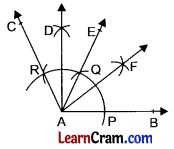Step 1: Draw a ray AR.
Step 2: Take A as centre and with suitable radius draw an arc which meets AB at P.
Step 3: Taking P as centre and with same radius, draw two arcs continuously, which meets the first arc at Q and R.
Step 4: Join AQ and AR such that ∠CAB = 120° and ∠EAB = 60°.
Step 5: Draw the bisectors AD and AF of ∠CAE and ∠EAR respectively. Then the measure of each angle is 30°.

Question 2.
Draw a line segment of 8 cm. Divide it into 4 equal parts using coin passes and ruler. R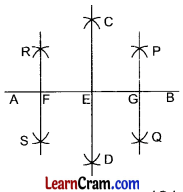Step 1: Draw a line AB = 8 cm.
Step 2: Draw two arcs with centre A and B and with radius A more than half of AB on opposite sides of it which intersect each other at C and D.
Step 3: Join CD which meets AB at E.
Step 2: Draw two arcs with centre A and B and with radius more than half of AB on opposite sides of it which intersect each other at C and D.
Step 3: Join CD which meets AB at E.
Step 4: Take E and F as centre and with suitable radius draw two arcs which intersect each other at P and Question
Step 5: Join PQ which meets AB at G.
Step 6: Take A and E as centres and with suitable radius draw two arcs which meet each other at R and S.
Step 7: Join R and S which meets AE at F.
Then AF = FE = EG = GB are four equal parts of AB.

Question 3.
Draw a circle of radius 4 cm with centre O. Draw two chords.
Name them AB and CD. Draw their perpendicular bisectors.
Do they pass through the centre of the circle?Step 1: Draw a circle of radius 4 cm and two chords AB and CD.
Step 2: Draw the perpendicular bisectors of AB and CD at E and F respectively.
Step 3: On producing the bisectors, they meet each other at centre O. Yes, they pass through the centre 0.Question 4.
Draw a line l and a point P outside the line. Draw a line through P and parallel to line l.